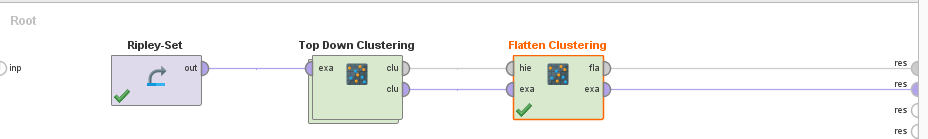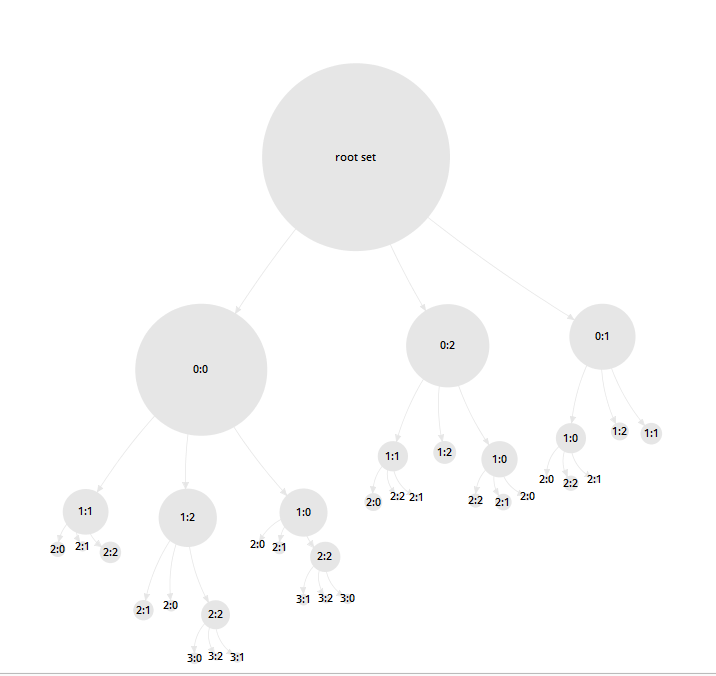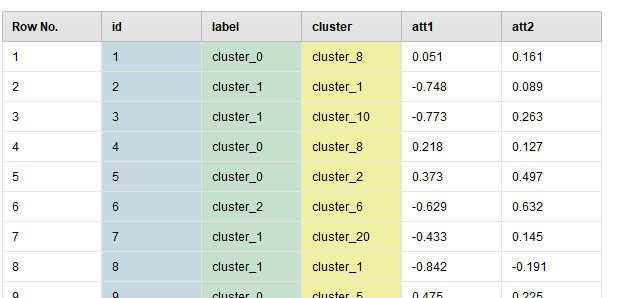# K means Clustering

Member Posts: 13Contributor I
edited March 2019 in Help
Hello,

I have a quick question, i am build 3 clusters based on RFM Score. R will represent the recent visit to branch , f will represent how often the customer visit within a year , and finally M will represent the amount of money occurs when the customer make a transaction once visit the branch.

once i create the 3 clusters: can re-cluster each cluster into several Clusters  based one some variables i choose ?

Thank you
Mario

Tagged:

• Administrator, Employee, RapidMiner Certified Analyst, RapidMiner Certified Expert, Member Posts: 363RM Data Scientist
Hi @mario_sark,

Are you building something like a hierarchical cluster model?

You can try the top-down clustering operator with flatten. But if you have any ground truth tags in the data, better go supervised.Your output data will have high-level grouping label and also low-level detailed cluster ID.```<?xml version="1.0" encoding="UTF-8"?><process version="9.2.000">
<context>
<input/>
<output/>
<macros/>
</context>
<operator activated="true" class="process" compatibility="9.2.000" expanded="true" name="Root" origin="GENERATED_TUTORIAL">
<parameter key="logverbosity" value="init"/>
<parameter key="random_seed" value="2001"/>
<parameter key="send_mail" value="never"/>
<parameter key="process_duration_for_mail" value="30"/>
<parameter key="encoding" value="SYSTEM"/>
<process expanded="true">
<operator activated="true" class="retrieve" compatibility="9.2.000" expanded="true" height="68" name="Ripley-Set" origin="GENERATED_TUTORIAL" width="90" x="112" y="34">
<parameter key="repository_entry" value="//Samples/data/Ripley-Set"/>
</operator>
<operator activated="true" class="top_down_clustering" compatibility="9.2.000" expanded="true" height="82" name="Top Down Clustering" origin="GENERATED_TUTORIAL" width="90" x="313" y="238">
<parameter key="create_cluster_label" value="true"/>
<parameter key="max_depth" value="5"/>
<parameter key="max_leaf_size" value="20"/>
<process expanded="true">
<operator activated="true" class="concurrency:k_means" compatibility="9.0.001" expanded="true" height="82" name="K-Means" origin="GENERATED_TUTORIAL" width="90" x="246" y="30">
<parameter key="remove_unlabeled" value="false"/>
<parameter key="k" value="3"/>
<parameter key="max_runs" value="10"/>
<parameter key="determine_good_start_values" value="false"/>
<parameter key="measure_types" value="BregmanDivergences"/>
<parameter key="mixed_measure" value="MixedEuclideanDistance"/>
<parameter key="nominal_measure" value="NominalDistance"/>
<parameter key="numerical_measure" value="EuclideanDistance"/>
<parameter key="divergence" value="SquaredEuclideanDistance"/>
<parameter key="kernel_gamma" value="1.0"/>
<parameter key="kernel_sigma1" value="1.0"/>
<parameter key="kernel_sigma2" value="0.0"/>
<parameter key="kernel_sigma3" value="2.0"/>
<parameter key="kernel_degree" value="3.0"/>
<parameter key="kernel_shift" value="1.0"/>
<parameter key="kernel_a" value="1.0"/>
<parameter key="kernel_b" value="0.0"/>
<parameter key="max_optimization_steps" value="100"/>
<parameter key="use_local_random_seed" value="false"/>
<parameter key="local_random_seed" value="1992"/>
</operator>
<connect from_port="example set" to_op="K-Means" to_port="example set"/>
<connect from_op="K-Means" from_port="cluster model" to_port="cluster model"/>
<portSpacing port="source_example set" spacing="0"/>
<portSpacing port="sink_cluster model" spacing="0"/>
</process>
</operator>
<operator activated="true" class="multiply" compatibility="9.2.000" expanded="true" height="103" name="Multiply" width="90" x="514" y="34"/>
<operator activated="true" class="flatten_clustering" compatibility="9.2.000" expanded="true" height="82" name="Flatten Clustering" width="90" x="648" y="238">
<parameter key="number_of_clusters" value="3"/>
<parameter key="remove_unlabeled" value="false"/>
</operator>
<connect from_op="Ripley-Set" from_port="output" to_op="Top Down Clustering" to_port="example set"/>
<connect from_op="Top Down Clustering" from_port="cluster model" to_op="Multiply" to_port="input"/>
<connect from_op="Top Down Clustering" from_port="clustered set" to_op="Flatten Clustering" to_port="example set"/>
<connect from_op="Multiply" from_port="output 1" to_port="result 1"/>
<connect from_op="Multiply" from_port="output 2" to_op="Flatten Clustering" to_port="hierarchical"/>
<connect from_op="Flatten Clustering" from_port="example set" to_port="result 2"/>
<portSpacing port="source_input 1" spacing="0"/>
<portSpacing port="sink_result 1" spacing="0"/>
<portSpacing port="sink_result 2" spacing="0"/>
<portSpacing port="sink_result 3" spacing="0"/>
</process>
</operator>
</process>
```
YY
• Member Posts: 13Contributor I
Hi @yyhuangyyhuang ,

Thank you for you reply ,

these my project Steps:
1- Calculate the RFM
2- Calculate the CP (Customer Power) and give a score
3 - Now i Have as fields : R, F, M, CP
4- Create clusters based on these Variables. (most Prob we want 3 or 4)
5- once we had these clusters we need to do further analysis on each cluster and extract more variables. (maybe 5 variables)
6- now i have more data about my customer in each Cluster. (these that i would use to apply the clustering technique again)

my question was if this is possible to be done. or I have another solution to achieve this Goal

Thank you Again,
Mario